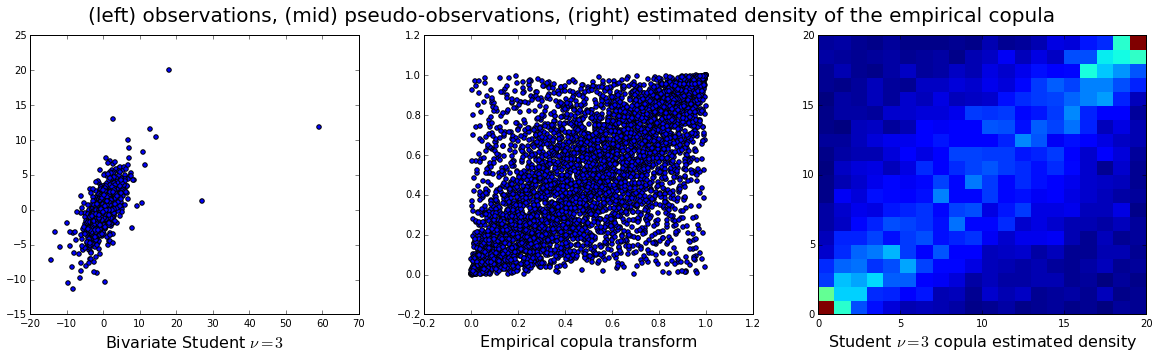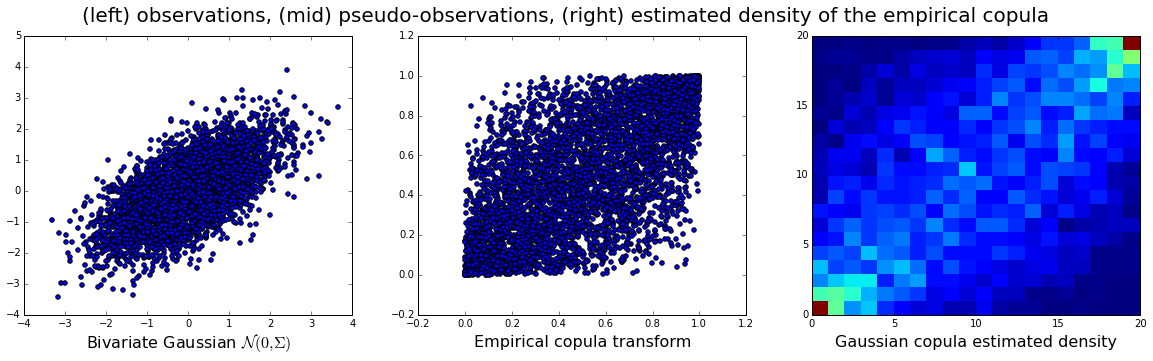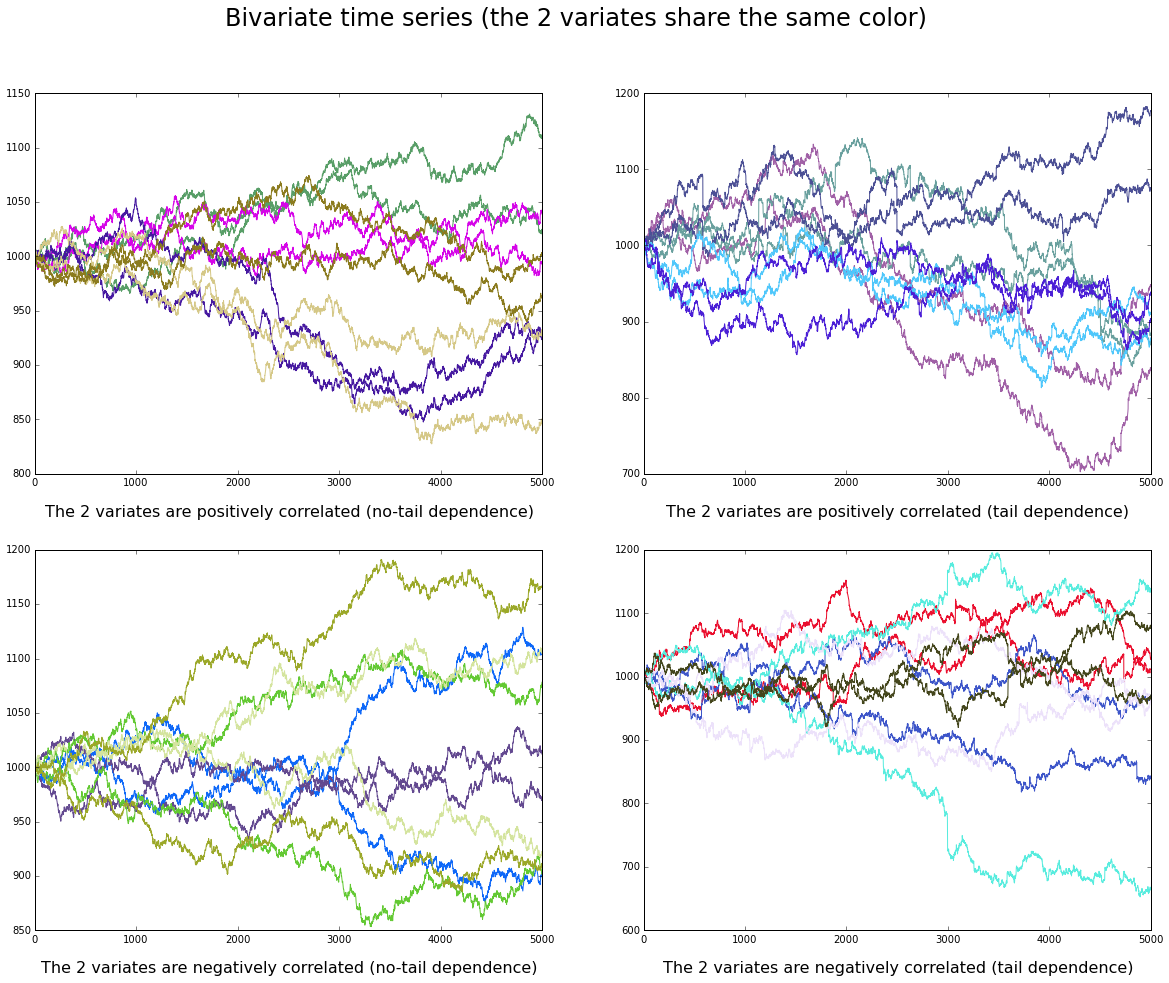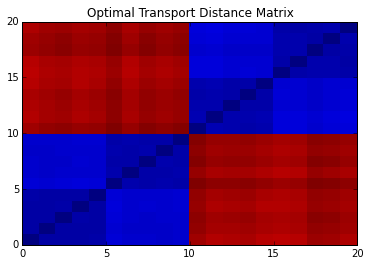# Tech Blog

## Our Data Scientists Comment on The Grapples

### Optimal Copula Transport - A tutorial

by Gautier MARTI, Hellebore Capital Management

In this tutorial (based on our paper), we present a new methodology for clustering multivariate time series leveraging optimal transport between copulas. Copulas are used to encode both (i) intra-dependence of a multivariate time series, and (ii) inter-dependence between two time series. Then, optimal copula transport allows us to define two distances between multivariate time series: (i) one for measuring intra-dependence dissimilarity, (ii) another one for measuring inter-dependence dissimilarity based on a new multivariate dependence coefficient which is robust to noise, deterministic, and which can target specified dependencies.

### How to build an empirical copula?

The empirical copula can be seen as the empirical distribution of the rank transformed data: To compute the empirical copula, one has to:

1. transform each variable x_i into a uniform u_i; it can be done by computing the ranks of the realizations, and normalize them into $[0,1]$:
u_i = scipy.stats.mstats.rankdata(x_i) / len(x_i)
2. estimate on $[0,1]^d$ its distribution.

From utils.py, we importe functions to generate random samples, and plot graphs.

In :
from utils import *
%matplotlib inline


First, we illustrate the empirical copula transform on a sample of $N = 5000$ observations drawn from a bivariate Student-t distribution.

The bivariate empirical Student-t copula is built from the $N = 5000$ observations drawn from the bivariate Student-t distribution of mean $\mu = 0$, scale $\Sigma = \begin{pmatrix} 1 & 0.65 \\ 0.65 & 1 \end{pmatrix}$ and degree of freedom $\nu = 3$:

In :
N = 5000
nbins=20
mean = [0,0]; cov = [[1,0.65],[0.65,1]]
XS,empirical_copula_XS,density_ecopula_XS,signatures_ecopula_XS = gen_sample(mean,cov,N,nbins,"Student")
plot_copula_sample(XS,empirical_copula_XS,density_ecopula_XS,"Student")### How to compute optimal transport between two copulas?

To illustrate the optimal transport distance (also called Earth Mover Distance), we generate another sample of $N = 5000$ observations from a bivariate Gaussian. Then, we will compute the distance between the empirical copula estimated from the Student-t realizations and the empirical copula estimated from the Gaussian realizations.

Below, we build the bivariate empirical copula from the $N = 5000$ observations drawn from the bivariate Gaussian distribution of mean $\mu = 0$ and covariance $\Sigma = \begin{pmatrix} 1 & 0.65 \\ 0.65 & 1 \end{pmatrix}$:

In :
from utils import gen_sample
mean = [0,0]; cov = [[1,0.65],[0.65,1]]
XG,empirical_copula_XG,density_ecopula_XG,signatures_ecopula_XG = gen_sample(mean,cov,N,nbins,"Gaussian")
plot_copula_sample(XG,empirical_copula_XG,density_ecopula_XG,"Gaussian")### Earth Mover Distance:

The Earth Mover Distance computes the work/cost to move dirt from one place to another. This cost depends on two quantities: how much dirt we have to move from one place to another, and how far are these places.

Based on this idea, we estimate a distance between two copulas by computing the work to transform one empirical copula density to another. In practice, the density on $[0,1]^d$ is estimated using a binning: each bin contains a mass corresponding to the frequency of realizations falling in this bin. Instead of using a "bin-to-bin" distance, the Earth Mover Distance allows to compare bins at different locations. The algorithm which computes the EMD distance will eventually match two similar bins at different locations if their ground distance is small enough to minimize the overall work. It helps to reduce undesirable binning side-effects.

Basically, the algorithm used to compute the EMD distance is the Hungarian algorithm. Computational complexity of the Hungarian algorithm is $\mathcal{O}(\text{nbins}^3)$, which is quite expensive for applications. Fast approximations have been developed recently to speed up the computations:

In :
from pyemd import emd
#the ground distance between bins
dist_mat = build_dist_mat(signatures_ecopula_XS)
#the mass of each bins
sig_S = np.array([signatures_ecopula_XS[i] for i in range(0,len(signatures_ecopula_XS))])
sig_G = np.array([signatures_ecopula_XG[i] for i in range(0,len(signatures_ecopula_XG))])
start = time.time()
dist_SG = emd(sig_S,sig_G,dist_mat)
end = time.time()
print("EMD between the Student and Gaussian copulas = "+str(dist_SG))
print("time: "+str(end-start)+" s")

EMD between the Student and Gaussian copulas = 0.016364698102008236
time: 0.19201898574829102 s


We can observe that computing such distances is indeed costly. But we will highlight in the next sections the modelling benefits of using them. We will illustrate potential applications of clustering with the optimal transport distance between copulas using time series data from DataGrapple. We also benchmark the approach against idealized situations where we know what is the generating process and what underlying structure is hidden in the data. We empirically show that we can recover it.

## Application: Optimal copula transport for clustering multivariate time series

### Intra-dependence similarity between time series

Suppose we have $N$ bivariate time series. We are interested in clustering these time series according to their intra-dependence, i.e. the dependence between their two coordinates.

For example, one entity can be represented by its:

1. (price, volume) time series
2. (bid, offer)-price time series
3. (equity, credit) time series
4. (short term, long term)-debt time series

Clustering based on this information can allow to discriminate between entities whose:

• large price moves are strongly correlated to high volumes vs. those which gap with low liquidity
• bid/offer are strongly correlated vs. those whose bid/offer is often anti-correlated due to uncertainty

and investigate the relations between equity & credit, and the debt term structure distortions.

Now, we present an idealized situation of the above examples. We simulate $20$ bivariate time series which represent $20$ assets characterized by $2$ characteristics, say equity price and credit price. For $10$ of the $20$ assets, credit and equity are anti-correlated: when credit price goes up, equity price usually goes down (these companies have to borrow money for surviving). For the other $10$ assets, credit and equity are correlated: when credit goes up, equity goes up (these companies are doing well, borrowing is for efficient investment). Furthermore, these two groups of size $10$ are subdivided into two more groups of size $5$ each: a group of assets whose credit/equity comovements undergo a standard correlation encoded by a bivariate Gaussian; the other group of assets exhibit credit/equity comovements undergoing tail dependence (they undergo violent comovements together) encoded by a bivariate Student-t with $\nu = 3$ degree of freedom.

To summarize, we have 20 bivariate time series:

• 10 with positive dependence, 5 normally distributed and 5 with tail-dependence,
• 10 with negative dependence, 5 normally distributed and 5 with tail-dependence.

A clustering procedure should first split the sample in two (positive vs. negative dependence), then each cluster is again divided in two (no tail-dependence vs. tail-dependence).

In :
N = 5000
timeseries = []
increments = []
#POSITIVE DEPENDENCE
mean = [0,0]; cov = [[1,0.65],[0.65,1]]
for i in range(5):
XG,empirical_copula_XG,density_XG,signatures_ecopula_XG = gen_sample(mean,cov,N,nbins,"Gaussian")
timeseries.append(signatures_ecopula_XG)
increments.append(XG)
for i in range(5):
Xsample,empirical_copula,density_ecopula,signatures_ecopula = gen_sample(mean,cov,N,nbins,"Student")
timeseries.append(signatures_ecopula)
increments.append(Xsample)
#NEGATIVE DEPENDENCE
mean = [0,0]; cov = [[1,-0.65],[-0.65,1]]
for i in range(5):
XG,empirical_copula_XG,density_XG,signatures_ecopula_XG = gen_sample(mean,cov,N,nbins,"Gaussian")
timeseries.append(signatures_ecopula_XG)
increments.append(XG)
for i in range(5):
Xsample,empirical_copula,density_ecopula,signatures_ecopula = gen_sample(mean,cov,N,nbins,"Student")
timeseries.append(signatures_ecopula)
increments.append(Xsample)

In :
draw_timeseries(increments)The $K = 4$ groups of $5$ bivariate time series described are displayed. Each of the $N = 20$ assets have a color that is shared by its two coordinates (credit and equity).

Now, we compute (using optimal transport) the distance matrix oct_dist between the $N = 20$ bivariate copulas encoding the intra-dependence of these bivariate time series:

In :
#the ground distance
dist_mat = build_dist_mat(signatures_ecopula)
#compute the EMD distance between each pair of copulas
Nex = len(timeseries)
oct_dist = np.zeros((Nex,Nex))
for i in range(Nex):
sig_i = np.array([timeseries[i][k] for k in range(0,len(timeseries[i]))])
for j in range(Nex):
if j > i:
sig_j = np.array([timeseries[j][k] for k in range(0,len(timeseries[j]))])
oct_dist[i,j] = emd(sig_i,sig_j,dist_mat)
oct_dist[j,i] = oct_dist[i,j]

plt.pcolormesh(oct_dist)
plt.title("Optimal Transport Distance Matrix")
plt.show()We can see that the $K = 4$ groups are blatant on the distance matrix displayed. The big blocks of size $10 \times 10$ represent the positive vs. negative intra-dependence groups. The 4 small blocks of size $5 \times 5$ subdividing them represent the no-tail vs. tail-dependence groups.

Applying a usual hierarchical clustering algorithm such as Average Linkage on the above distance matrix will recover the positive vs. negative dependence clusters, and then the no-tail vs. tail-dependence clusters.

### Application to CDS data: clustering CDS single-names based on their (3yr,10yr) dependence

In this section, we apply the methodology to 'real' data: credit default swaps' term structures. Each credit default swap (CDS) is represented by a bivariate time series: the price of insurance for 3 years protection against default of the underlying company, and the price of insurance for 10 years protection against the same underlying company.

We want to test if there are groups of CDS that share the same intra-dependence between their 3-year term and their 10-year term.

We report below blatant groups that we have found, and a representative empirical copula characterizing the intra-dependence common to these CDS. We can notice that their 3-year and 10-year time series are strongly dependent, with some tail-dependence (red bins in the corner).

Cluster "European Banks": Crédit Agricole, Barclays Bank, Banco Bilbao Vizcaya Argentaria, BNP Paribas, Commerzbank, Deutsche Bank, Intesa Sanpaolo, Lloyds Bank, The Royal Bank of Scotland, Banco Santander, Société Générale, UBS, Unicredit.Cluster "European Insurers": Aegon, Allianz, Assicurazioni Generali, Aviva, Axa, Hannover Rueck, Münchener Rückversicherungs-Gesellschaft, Swiss Reinsurance Company.There is also a singleton cluster: Zurich Insurance Company. This one has a very strong positive dependence between its short term (3-year) and its long term (10-year) CDS.On the contrary, the dependence between 3yr and 10yr prices seems much lower on the other European industries.### Inter-dependence: how to target specific dependence?

In this section, we present another use of the optimal transport distance between copulas. Now, we want to qualify and quantify inter-dependence, i.e. the comovements between distinct objects rather than measuring whether distinct objects have the same dependence between their respective coordinates.

We can use the presented distance between copulas to define a novel dependence coefficient which can target specified dependencies.

To do so

1. Fit an empirical copula to the data, and estimate its density $C$
2. Build the density of the independence copula $C_{\text{ind}}$ which is uniform on $[0,1]^d$
3. Build the densities for the different target-dependencies $C_i$; for instance, perfect positive dependence is represented by uniform mass on the cube's diagonal
4. Compute the Target Dependence Coefficient as defined below: $$\text{TDC} = \frac{\mathrm{dist}(C_{\mathrm{ind}},C)}{\mathrm{dist}(C_{\mathrm{ind}},C) + \min_i \mathrm{dist}(C,C_i)}$$

In the bivariate case depicted below, the target-dependencies are perfect positive dependence and perfect negative dependence. In this example, the data is nearer to "perfect positive dependence" than to "perfect negative dependence".We can readily specify other dependence targets to our coefficient. For example, we run a benchmark code strongly inspired from David Lopez-Paz experiments for his NIPS, 2013 paper.

In this experiment, we compare our coefficient TDC (whose power is represented by the deep-blue curve in the graph below) to state-of-the-art dependence coefficient. This experiment aims at measuring the power of a dependence measure, i.e. whether it can discern between dependent and independent samples.

The x-axis measures the noise added to the sample, the y-axis the power of the dependence coefficient estimated by the frequency the coefficient is able to discern between the dependent sample and the pure noise (independent one). The experiment is led for several dependence-pattern.

As a basic check, we verify that no coefficient can discern between the "dependent" sample (with no dependence (row 2, col 2)) and the independent sample.

We notice that our coefficient TDC performs at least as well as the other coefficients, if not much better for complex patterns (cf. (row 4, col 2)).

The R code that can produce the illustration below is available in the .zip file at the end of the tutorial.### Inter-dependence: how far from the perfect positive dependence?

In this section, we consider 'univariate' time series such as the 5-year CDS spreads of single-names as they can be seen at DataGrapple with the Grapple "CDS Time Series". But instead of computing a correlation coefficient (such as Pearson, Spearman or Kendall), we apply the presented methodology and use the Target Dependence Coefficient with "perfect positive dependence" as the only target. With this methodology, we should keep more information from the copula than using the other coefficients.

#### Some bivariate empirical copulas computed from a few pairs of 5yr CDS time series:

European banks are strongly dependent. For example, BNP Paribas 5yr CDS vs. Société Générale 5yr CDS empirical copula:Western European sovereigns are also strongly positvely correlated. However, we can notice that when stressed, they tend to be negatively correlated (an anti-diagonal is apparent on the copula).European and Japanese corporates are nearly independent. Here Anglo American 5yr CDS (mining company, producer of diamonds, copper, nickel, iron ore, coal) vs. Kobe Steel 5yr CDS (steel manufacturer, importing/exporting iron ore and coal).#### Comparing the clustering obtained using Pearson vs. Spearman vs. Kendall vs. Target Dependence Coefficient between the 5-year CDS

We compare below the impact of the choice of a particular dependence coefficient on the clustering of financial time series.

Following Mantegna's seminal work, most of the researchers clustering financial time series use the Pearson correlation coefficient on the log-returns. This coefficient is quite brittle to outliers and heavy-tailed distribution marginals, thus not very robust when applied to financial time series.

On the contrary, rank-based dependence measure such as Spearman $\rho_S$, Kendall $\tau$ correlation coefficient (and the Target Dependence Coefficient) are known to be much more robust to these perturbations. Indeed, they benefit from the properties of copulas. More precisely, let $X,Y$ be random variables and $C$ the copula of $(X,Y)$ joint distribution, the following relations hold:

$$\tau(X,Y) = 4 \int\int_{[0,1]^2} C(u,v) dC(u,v) - 1$$$$\rho_S(X,Y) = 12 \int\int_{[0,1]^2} C(u,v)dudv - 3$$

In particular, the last expression can be rewritten

$$\rho_S(X,Y) = 12 \int\int_{[0,1]^2} \left( C(u,v) - uv\right) dudv,$$

which is a (signed) distance (in volume) between the copula $C$ and the independence copula $C_{\mathrm{ind}} = uv$.

The Target Dependence Coefficient is also partly a distance to the independence copula (density), but incorporates the target-copulas.

The clustering results obtained on the CDS time series illustrate these relationships between the dependence coefficients.

How to read the graph: The graph below conveniently displays the clustering results. Each of the four columns corresponds to a clustering obtained using the four different coefficients. Each rectangle represents a cluster. Hovering over the cluster tells you how many CDS are in this cluster (the rectangle size is proportional to the number of CDS in the corresponding cluster). Hovering over an edge between two clusters tells you which CDS are shared by these two clusters. If two clusterings were perfectly similar, then there would be a one-to-one mapping between their clusters. If many edges cross and split between two clusterings, it readily tells you that the two clusterings are much dissimilar.

We can observe that the clustering obtained from using Pearson (standard linear) correlation is much different from the others.

The clusterings obtained from using Spearman and Kendall coefficients are much similar (as expected).

The clustering obtained from TDC is more "balanced", but still quite similar to the Spearman and Kendall ones.

• We can notice that in this case the cluster of "Asian Ex-Japan" CDS is splitted into two clusters: Korean & China companies vs. Australian companies.
• The European cluster is splitted in two clusters.
• The US clusters are different.

The Spearman, Kendall, TDC clusterings agree on:

• The European Sovereigns cluster,
• The Japanese companies cluster.
In :
%%html
<div>
<script src="javascripts/d3.min.js"></script>
<script src="javascripts/sankey.js"></script>
<center><big><strong>Hierarchical Clustering Mapper - HCMapper</strong></big></center>
<center>Pearson, Spearman, Kendall, Target Dependence Coefficient</center>
<p id="chart">
<script src="javascripts/mix_graph.js"></script>
</div>

Hierarchical Clustering Mapper - HCMapper
Pearson, Spearman, Kendall, Target Dependence Coefficient